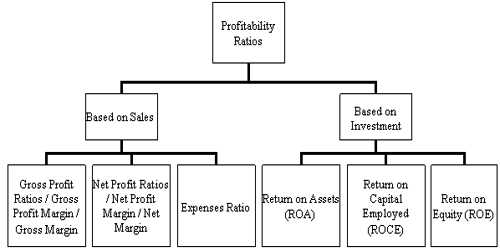Accounting

# Profitability RatiosProfitability Ratios

The efficiency of a business is measured by profitability. Profitability ratio measures the profit earning capacity of the business concern. A profitability ratio is determined by profitability, which is a technique to evaluate a company’s performance. Profitability is basically the competence to make a profit, and a profit is what is left over from income earned after you have deducted all costs and expenses related to earning the income.

The important profitability ratios are discussed below:

1. Gross Profit Ratio
2. Net Profit Ratio
3. Operating Profit Ratio
4. Operating Ratio

Gross Profit Ratio

This ratio indicates the efficiency of trading activities. The relationship of Gross profit to Sales is known as gross profit ratio. The ratio is calculated as:

Gross Profit Ratio = (Gross Profit / Sales) x 100

Gross profit is taken from the Trading Account of a business concern. Otherwise, Gross profit can be calculated by deducting the cost of goods sold from sales. Sales means, Net sales.

Gross Profit = Sales – Cost of goods sold

Cost of goods sold = Opening Stock + Purchases – Closing Stock

or, Sales – Gross Profit

Net Profit Ratio

This ratio determines the overall efficiency of the business.   The relationship of Net profit to Sales is known as net profit ratio. The ratio is calculated as:

Net Profit Ratio = (Net Profit/ Sales) x 100

Net profit is taken from the Profit and Loss account of the business concern or the gross profit of the concern less administration expenses, selling and distribution expenses and financial expenses.

Operating Profit Ratio

This ratio is an indicator of the operational efficiency of the management. It establishes the relationship between Operating profit and Sales. The ratio is calculated as:

Profit Ratio = (Operating Profit Operating/Sales) x 100

Where operating profit is Net profit + Non-operating expenses – Non-operating income.

Where Non-operating expenses are interest on loan and loss on sale of assets.

Non-operating incomes are the dividend, interest received, and profit on the sale of the asset.

or, Operating profit = Gross profit  – Operating expenses.

Operating expenses include administration, selling and distribution expenses. Financial expenses like interest on the loan are excluded for this purpose.

Operating Ratio

This ratio determines the operating efficiency of the business concern. Operating ratio measures the amount of expenditure inured in production, sales, and distribution of output. The relationship between

Operating cost to Sales is known as Operating Ratio. The ratio is calculated as:

Operating Ratio = (Cost of goods sold + Operating expenses)/Sales x 100